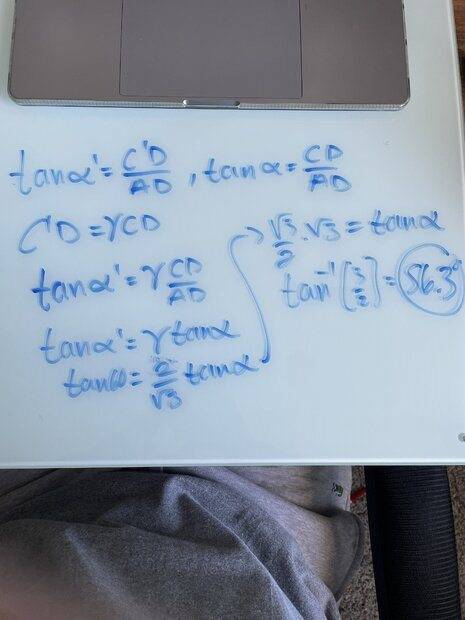# Find the contraction of angles seen by an observer

Homework Helper
Gold Member
You are getting very close. But you are making some careless errors regarding which length is the contracted length and the definition of gamma.

I also know that C'D = ϒCD, which is the contracted length is equal to the uncontracted length times gamma.
I know that gamma is equal to √(¾) is is √(3)/2 for this problem.
The relation C'D = ϒCD is correct. But C'D is the uncontracted length and CD is the contracted length.

Gamma is not equal to ##\sqrt{1-v^2/c^2}##. Gamma is ##\large \frac{1}{\sqrt{1-v^2/c^2}}##

•PeroK
guyvsdcsniper
You are getting very close. But you are making some careless errors regarding which length is the contracted length and the definition of gamma.

The relation C'D = ϒCD is correct. But C'D is the uncontracted length and CD is the contracted length.

Gamma is not equal to ##\sqrt{1-v^2/c^2}##. Gamma is ##\large \frac{1}{\sqrt{1-v^2/c^2}}##